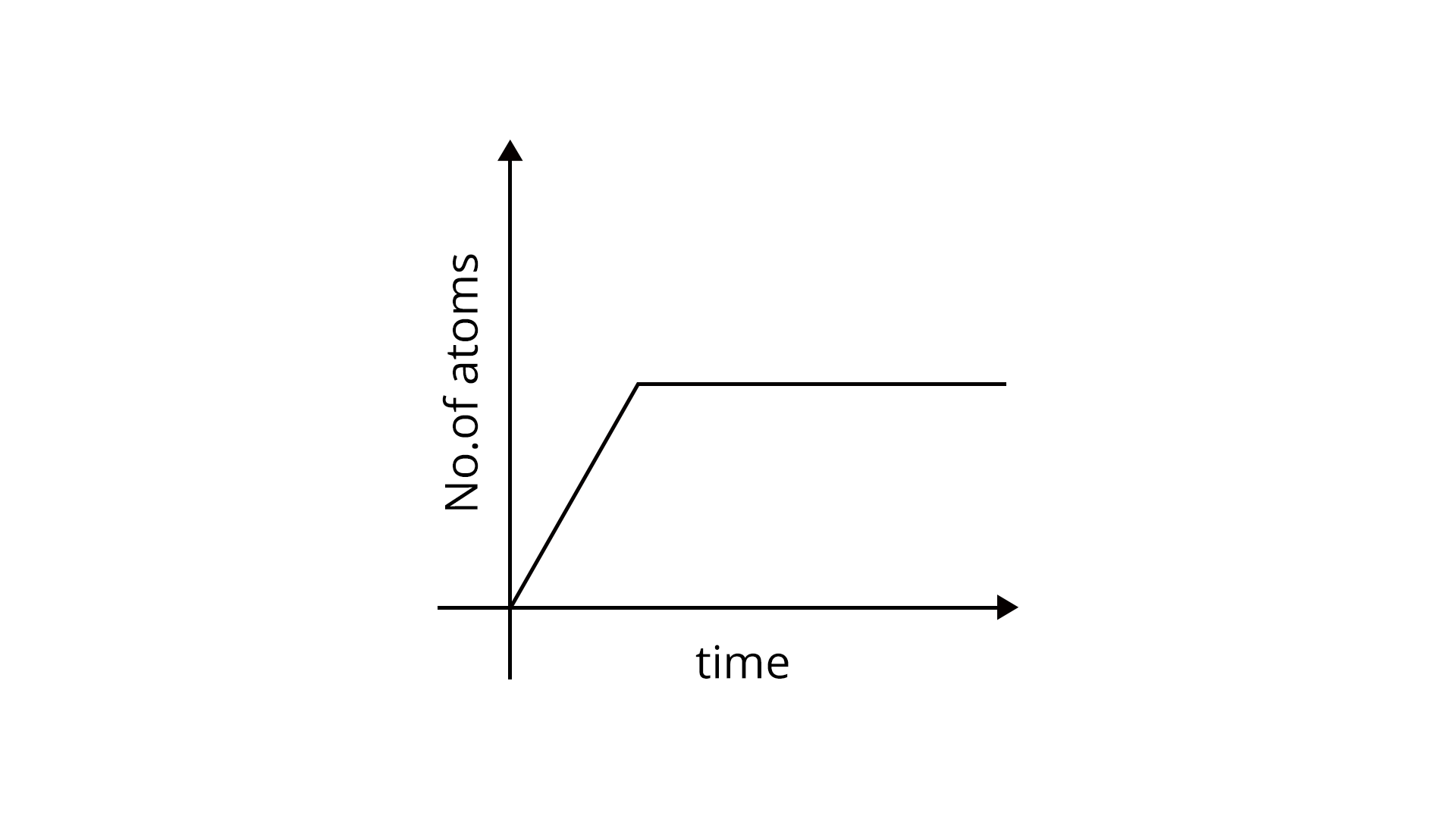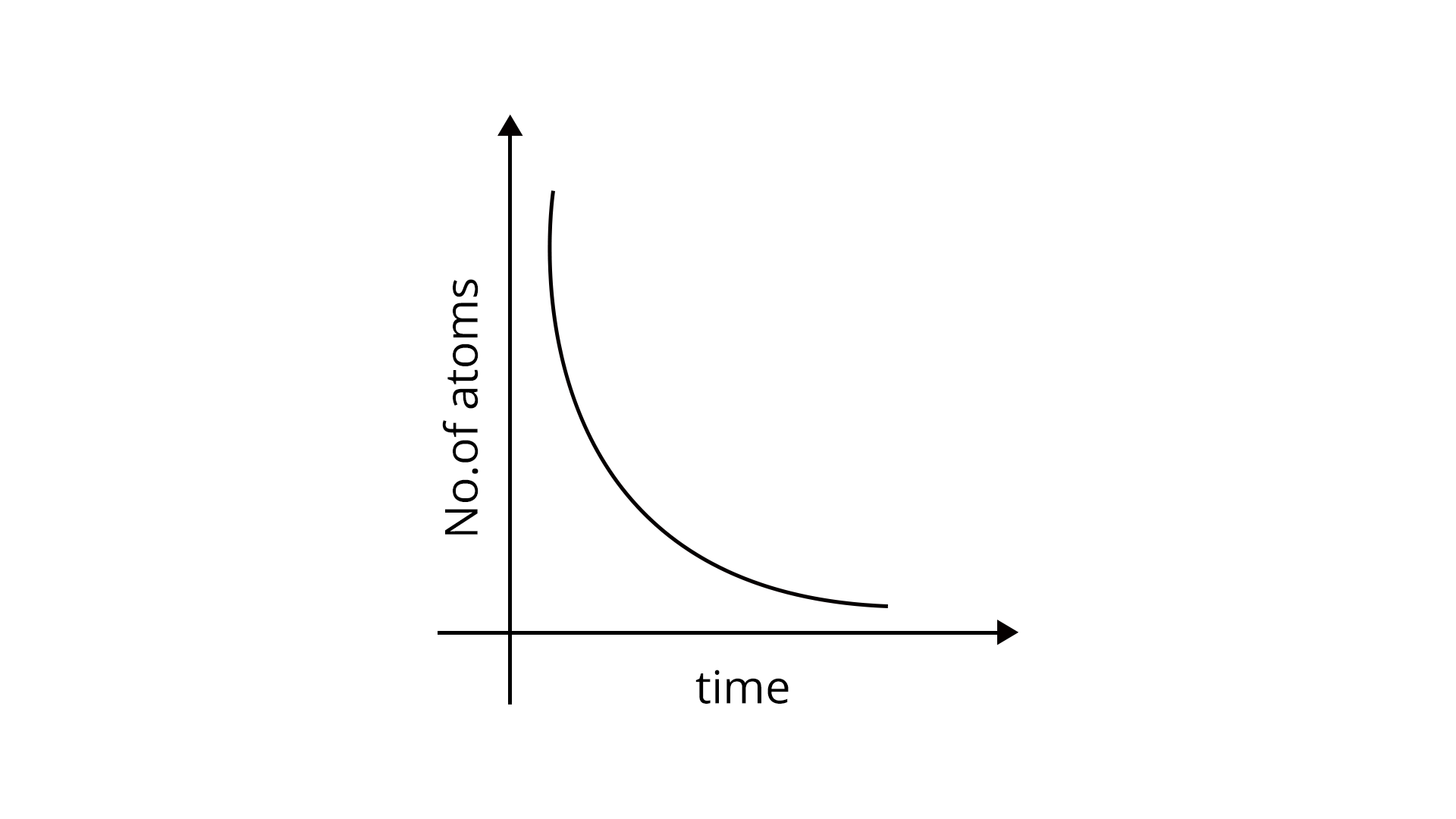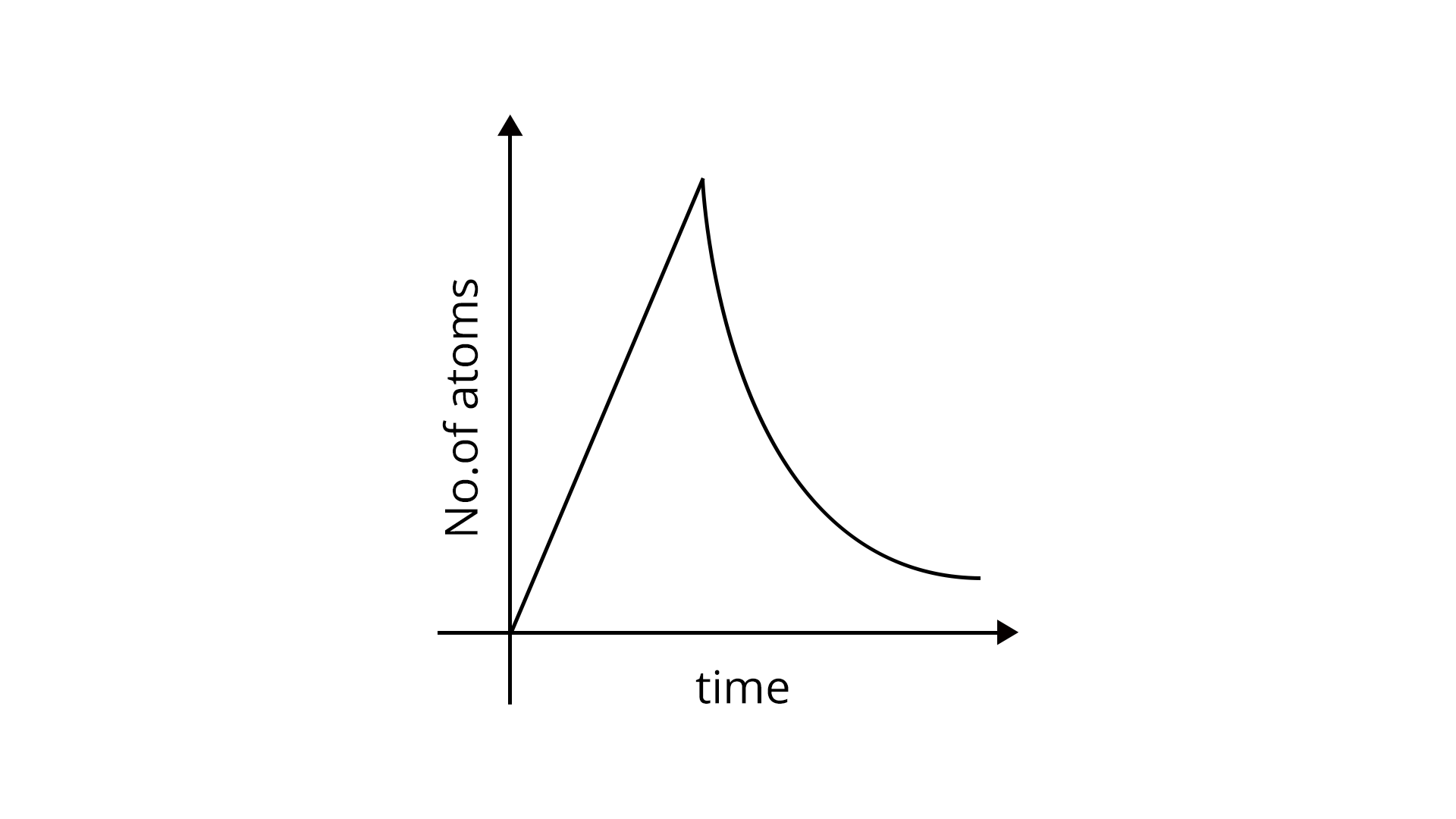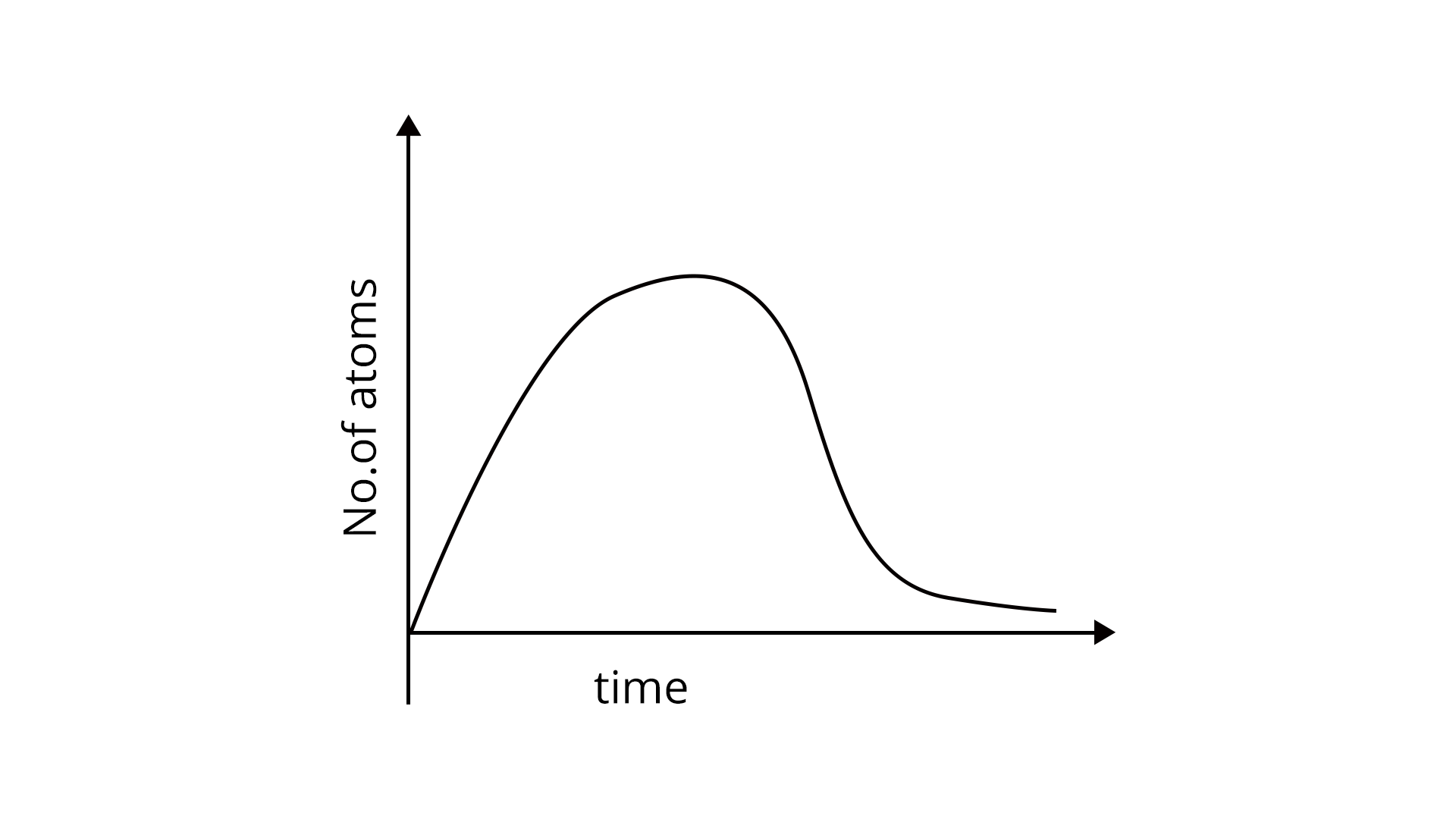Courses
Courses for Kids
Free study material
Free LIVE classes
More

# JEE Important Chapter - Atoms and NucleiLIVE
Join Vedantu’s FREE Mastercalss

## Important Concept of Atoms and Nuclei for JEE

The chapter Atoms and Nuclei gives us the idea about studying the structure of atoms and nuclei. This chapter is divided into two subcategories, the first one is atoms in which the concepts like Rutherford’s atom model, atomic spectra, Bohr’s model of hydrogen atom and energy level diagram have been discussed. The second one consists of concepts like nuclear size, nuclear density, nuclear binding energy, radioactivity and nuclear fission and fusion.

Now, let's move on to the important concepts and formulae related to JEE and JEE main physics atoms and nuclei exam along with a few solved examples.

## JEE Main Physics Chapter-wise Solutions 2022-23

### Important Topics of Atoms and Nuclei

• Rutherford's atomic model and its limitation

• Bohr model of hydrogen atom

• Bohr’s explanation of spectral series of hydrogen atom

• Nuclear size and nuclear density

• Mass energy relation and nuclear binding energy

• Alpha, beta and Gamma decay

• Nuclear fission and fusion

### Atoms and Nuclei Important Concept for JEE

 Name of the concept Key points of the concepts 1. Rutherford's atomic model and its limitation Every atom consists of a tiny central core, called the atomic nucleus in which the entire positive charge and the almost entire mass of the atom are concentrated.The atom as a whole is electrically neutral and electrons revolve around the nucleus in various circular orbits.As the electron revolves so it must lose energy continuously, it must spiral inwards and eventually fall into the nucleus. Therefore, atoms should emit a continuous spectrum but what we observe is a line spectrum. So this point isn’t explained by the Rutherford model which is the biggest limitation of this model. 2. Bohr’s model of hydrogen atom Bohr gives three postulates;Every atom consists of a central core called the nucleus which contains the positive charge and electrons revolve around it in their circular orbits.Electrons can revolve only in certain discrete non radiating orbits, called stationary orbits for which the total angular momentum of the revolving electron is an integral multiple of $\dfrac{h}{2\pi}$, where $h$ is the Planck’s constant.The emission or absorption of energy occurs only when an electron jumps from one of its specified orbits to another. The difference in the total energy of electrons in the two permitted orbits is absorbed when the electron jumps from the inner to the outer orbit. 3. Bohr’s explanation of spectral series of hydrogen atom Bohr postulated that the Lyman series is obtained when an electron jumps to the first orbit from any orbit. It lies in the ultraviolet region.Balmer series is obtained when an electron jumps to a second orbit from any outer orbit. It lies in the visible spectral region.The Paschen series is obtained when an electron jumps to the third orbit from any outer orbit. It lies in the infrared region.Brackett series is obtained when an electron jumps to the fourth orbit from any outer orbit. Pfund series is obtained when an electron jumps to the fifth orbit from any outer orbit. 4. Nuclear size and nuclear density A nucleus' volume is proportional to its mass number.The density of the nucleus matter is the ratio of the mass of the nucleus and its volume. Its value is $2.29 \times 10^{17}\, kg/m^3$. 5. Mass energy relation and nuclear binding energy Mass energy relation tells us that when a certain mass ($m$) disappears then an equivalent amount of energy $E$ appears and vice-versa.The energy with which nucleons in a nucleus are bonded is referred to as the nucleus' binding energy. It is determined by the amount of effort necessary to detach the nucleons from the nucleus at an infinite distance. 6. Radioactivity Radioactivity is the process by virtue of which a heavy element disintegrates itself without being forced by any external agent to do so.According to laws of radioactive disintegration, the number of atoms disintegrating per second at any instant is directly proportional to the number of radioactive atoms actually present in the sample at that instant.$-\dfrac{dN}{dt}\varpropto N$The half-life of a radioactive element is the amount of time it takes for half of the atoms present in the sample to decay.The average lifetime of the element is obtained by calculating the total lifetime of all the atoms of the element and dividing it by the total number of atoms present initially in the sample of the element 7. Alpha, beta and Gamma decay Alpha decay is the phenomenon of the emission of an alpha particle from a radioactive nucleus. Ex:$U^{238}_{92}\longrightarrow Th^{234}_{90}+He^4_2$Beta decay  is the phenomenon of emission of an electron from a radioactive nucleus. Ex:$Th^{234}_{90}\longrightarrow Pa^{234}_{91}+e^0_{-1}$Gamma decay is the phenomenon of emission of gamma-ray photons from a radioactive nucleus. Ex:$Ni^{60*}_{28}\longrightarrow Ni^{60}_{27}+E_{\gamma}$ 8. Nuclear fission and fusion Nuclear fission is the process of splitting a heavy nucleus (usually A>230) into two or more lighter nuclei.Nuclear fusion is the process by which two or more lighter nuclei combine to produce a single heavy nucleus.

### List of Important Atoms and Nuclei Formulas

 S.No. Name of the Concept Formula Rutherford's atomic model According to Rutherford’s model, the total energy of the electron orbit is, $E=\dfrac{e^2}{8\pi \epsilon_o r}$ Bohr’s model of hydrogen atom From 1st postulate of Bohr’s model of atom we can write;$\dfrac{mv^2}{r}=\dfrac{KZe^2}{r^2}$Here, $v$= velocity with which electron is moving and $r$= radius of the orbit.From 2nd postulate,$mvr=\dfrac{nh}{2\pi}$From 3rd postulate,$E_2-E_1=nh\nu$The electron's frequency in Bohr's stationary orbit is,$\nu=\dfrac{KZe^2}{nhr}$Total energy of electron in Bohr’s stationary orbit is,$E=-\dfrac{13.6}{n^2}eV$ Bohr’s explanation of spectral series of hydrogen atom The expression of wave number ($\overline{\nu}$) of radiation emitted when electron jumps from one orbit to another is,$\overline{\nu}=RZ^2[\dfrac{1}{n^2_1}-\dfrac{1}{n^2_2}]$In the case of hydrogen, $Z=1$ and for different types of series of H-atoms we have different values of $n_1$ and $n_2$. Nuclear size and nuclear density Relation between radius ($R$) and mass number($A$) of the nucleus,$R=R_oA^{1/3}$Here, $R_o$ is the constant having a value of $1.2 \times 10^{-15}\,m$. Mass energy relation and nuclear binding energy Mass energy relation is given as,$E=mc^2$Binding energy of the nucleus,$B.E.=[Zm_H+(A-Z)m_n-m(X_Z^A)]c^2$Here, $Z$= charge number, $A$= mass number, $m_n$ = mass of the proton, $m_n$ = mass of the neutron and $m_n$ = mass of nucleus $X_Z^A$. Radioactivity According to the law of radioactive decay, $-\dfrac{dN}{dt}\varpropto N$$R=-\dfrac{dN}{dt}= \lambda N$            Here, $\lambda$ =disintegration                          constant.Half life ($T_{1/2}$)of radioactive element is,$T_{1/2}=\dfrac{0.693}{\lambda}$Average life ($\tau$) is,$\tau= \dfrac{1}{\lambda}$The activity of an element is given as, $A=A_o e^{-\lambda t}$

### Difference Table Between Nuclear Fission and Fusion

 Nuclear Fission Nuclear Fusion Nuclear fission occurs when the nucleus of an atom breaks into lighter nuclei as a result of a nuclear reaction. Nuclear fusion is a reaction that happens when two or more light nuclei collide, resulting in the formation of a heavier nucleus. A great quantity of energy is produced when each atom splits. The amount of energy released during nuclear fusion is many times more than the amount of energy released during nuclear fusion. Fission reactions do not occur spontaneously in nature. Stars and the sun undergo fusion processes. A fission process requires little energy to split an atom. In a fusion reaction, a large amount of energy is required to fuse two or more atoms together. The principle of nuclear fission supports the operation of an atomic weapon. The hydrogen bomb works in the same way as a nuclear fusion weapon.

### Solved Examples

1. Calculate the number of revolutions per second done by a hydrogen atom electron in the third Bohr orbit.

Sol:

Given that, Bohr orbit ($n$) = 3

We have to calculate the frequency i.e, $\nu$.

In order to calculate the frequency of revolution we have to use the second postulate of Bohr’s atomic model according to which angular momentum of the electron should be equal to the integral multiples of $\dfrac{h}{2 \pi}$.

According to the second postulate of Bohr’s atomic model,

$mvr=\dfrac{nh}{2\pi}$

As we know that $v=\omega r$, so after putting this in the above equation we get,

$m\omega r^2=\dfrac{nh}{2\pi}$

$m (2\pi \nu) r^2=\dfrac{nh}{2\pi}$...........(As $\omega=2\pi \mu$)

$\nu = \dfrac{nh}{4\pi^2 m r^2}$

Now after putting the values of mass of electron($m=9.1 \times 10^{-31}\,kg$), Planck’s constant($h=6.6 \times 10^{-34}\, J/s$, radius of orbit($r=0.53 \times 10^{-10}\,m$) and $n=3$, we get;

$\nu = \dfrac{3 \times 6.6 \times 10^{-34}}{4 \times (3.14)^2 \times 9.1 \times 10^{-31} (0.53 \times 10^{-10})^2}$

On further simplification we get;

$\nu = \dfrac{19.8 \times 10^{-34} }{100.81 \times 10^{-51}}$

$\nu = 0.1964 \times 10^{17}\,rev/sec$

$\nu = 1.964 \times 10^{16}\,rev/sec$

Hence, the frequency of revolution done by an electron in the third orbit of a hydrogen atom is $1.964 \times 10^{16}\,rev/sec$.

Key point: The knowledge of the second postulate in Bohr’s atomic model is necessary to solve this problem.

1. A radioactive substance's mean life is 1500 years for alpha emission and 300 years for beta emission, respectively. Calculate the time it takes for three-quarters of a sample to decay if it is decaying by both alpha and beta emission at the same time. (Take, $\log_{10}4= 1.386$)

Sol:

Given that,

Mean life of alpha emission, $\tau_\alpha$ = 1500 years

Mean life of beta emission, $\tau_\beta$ = 300 years

Number of nuclei remains, $N={N_o} - \dfrac{3}{4}{N_o}=\dfrac{N_o}{4}$

To find: Time during which it decay to three fourths of a sample i.e, $t$.

To solve this problem we have to apply the concept of mean life time and also the laws of disintegration of radioactive elements. If we consider the $\lambda_\alpha$ and $\lambda_\beta$ be the decay constants of the alpha and beta emission then using the formula of mean life time, we can write;

$\lambda_\alpha=\dfrac{1}{\tau_\alpha}=\dfrac{1}{1500}$

And, $\lambda_\beta=\dfrac{1}{\tau_\beta}=\dfrac{1}{300}$

Total decay constant is given as,

$\lambda=\lambda_\alpha+\lambda_\beta$

$\lambda= \dfrac{1}{1500}+\dfrac{1}{300}$

$\lambda=\dfrac{6}{1500}=\dfrac{1}{250}\,yr^{1}$

Now, from laws of disintegration we can write,

$N=N_o e^{-\lambda t}$

After putting the values of $N$ , we get;

$\dfrac{N_o}{4}=N_o e^{-\lambda t}$

$t= \dfrac{\log_{e}4}{\lambda}$

Now after putting the value of $\lambda$, we get;

$t= 2.3026 (\dfrac{\log_{10}4}{1/250})$

$t = 2.3026 \times 1.386 \times 250$

$t = 797.8$ years

Hence, the time taken by the sample to decay its three fourths value both by alpha and beta emission is 797.8 years.

Key point: The concept of mean life time of a radioactive sample and laws of radioactive disintegration is important to solve this problem.

### Previous Year Questions from JEE Paper

1. There are $10^{10}$ radioactive nuclei in a given radioactive element, its half-life time is 1 minute. How many nuclei will remain after 30 seconds? (Take, $\sqrt{2}=1.414$) (JEE Main 2021)

a. $7 \times 10^{9}$

b. $2 \times 10^{9}$

c. $4 \times 10^{10}$

d. $10^{5}$

Sol:

Given that,

Original number of nuclei, ${N_o} = 10^{10}$

Half- life time, $t_{½} = 60\,s$

To find: Number of nuclei remain after time ($t$ = 30 seconds) i.e, $N$.

To solve this problem we have to use the concept of half life and also the relation of the number of nuclei decayed to the original number of nuclei present in the sample of a radioactive element.

Now using the concept of half live, we can write the relation between the number of nuclei decayed ($N$) to the original number of nuclei($N_o$) as,

$\dfrac{N}{N_o}=(\dfrac{1}{2})^{t/t_{1/2}}$

Now after putting the values of the quantities in the above relation, we get;

$\dfrac{N}{10^{10}}=(\dfrac{1}{2})^{30/60}$

$\dfrac{N}{10^{10}}=(\dfrac{1}{2})^{1/2}$

$N= \dfrac{10^{10}}{\sqrt{2}} \approx 7 \times 10^{9}$

Hence, the number of nuclei that remain after 30 seconds is $7 \times 10^{9}$. Therefore, option a is correct.

Key point: The application of the half life concept and the relation between the number of nuclei decayed to the original number of nuclei in a sample is important to solve this problem.

1. A sample of a radioactive nucleus A disintegrates to another radioactive nucleus B, which in turn disintegrates to some other stable nucleus C. Plot of a graph showing the variation of number of atoms of nucleus B versus time is :

(Assume that at t = 0, there are no B atoms in the sample) (JEE Main 2021)a.b.c.d.

Sol:

Given that, initially the number of atoms of $B=0$ at $t=0$ and the last product of reaction i.e, nuclei $C$ is a stable one. Now we have to find the behaviour of $B$ with respect to time as it decays to $C$.

To answer this question we have to use the concept of growth and decay of a radioactive sample. As it is mentioned that the number of $B$ atoms are zero at $t=0$ so the graph must start from the origin. When the rate of decay of B equals the rate of production of B, the number of atoms of B begins to increase and reaches a maximum value. Because growth and decay are both exponential functions, the number of atoms will start to decrease after that maximum value, hence the best possible graph is (d). Therefore, option d is correct.

Key point: The concept of growth and decay of a radioactive sample is essential to solve this problem.

### Practice Questions

1. Which level of doubly ionised lithium has the same energy as the ground state energy of a hydrogen atom? It is necessary to compare the orbital radii of the two levels. (Ans: 3,3)

2. The decay constant for the radioactive nuclide $Cu^{64}$ is $1.5 \times 10{-5}\,s{-1}$. Find the activity of a sample containing $1\,\mu g$ of $Cu^{64}$. The atomic weight of copper is equal to 63.5 g/mole. The mass difference between the supplied radioisotope and regular copper is ignored.(Ans: 3.87 Ci)

### Conclusion

In this article we have discussed the various models related to the structure of atoms and nuclei like Rutherford’s model of atom and Bohr’s model of atom. Bohr's model of atoms is widely accepted because it explained properly about the line spectra of hydrogen atoms which were not explained by Rutherford's model of atoms. We have also mentioned the various important atoms and nuclei formulas. We have also discussed topics like nuclear size, nuclear density, radioactivity, mass-energy relation and binding energy of nuclei.

## Important Related Links for JEE Main 2022-23

Last updated date: 26th Sep 2023
Total views: 2.7k
Views today: 0.02k

## FAQs on JEE Important Chapter - Atoms and Nuclei

1. What is the weightage of the Atoms and Nuclei in the JEE exam?

This chapter comes under the branch of Modern physics which ultimately leads to approximately 1-2 questions every year. Thus, the total weightage of this chapter is approximately 2-3 % in the exam.

2. What is the level of difficulty of the questions from the Atoms and Nuclei chapter?

As this chapter comes under Modern physics so the difficulty level of the questions asked in this chapter is quite high. As this chapter deals with the modern edge of Physics’s research and development, therefore it is important to study the chapter for the exam rather than skipping it.

3. Is it genuinely advantageous to revise previous year's Atoms and Nuclei chapter questions for this exam?

In order to score well and become familiar with the exam's difficulty level, we must practise the previous year's problems. It boosts our self-esteem while simultaneously exposing us to areas where we may improve. Solving past ten to fifteen-year question papers will help you better understand a concept and can also show you how many times a concept or topic will be repeated in the test. It is also good to prepare for the atoms and nuclei jee notes by practising the previous year's problems.